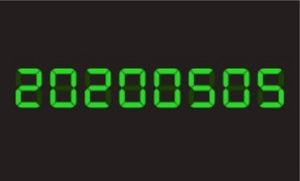# Happy Math Day ! 5/5 （鏡像日、福哈伯、連續整數次方和）

### Amathing Number

• 月份、日期都是五邊形數。
• 5/5旋轉180度還是5/5。
• 月份、日期都是質數，而且都可以寫成兩個質數的和。
• 2020年的5/5更特別，是鏡像日。
拿片鏡子放在兩個零中間，會發現有趣的事喔！順帶一題，這種顯示方式叫做Segment Display。

### Amathing Person$1^p+2^p+3^p+\cdots +n^p$$a=1+2+3+\cdots+n=\frac{n(n+1)}{2}$$1^3+2^3+3^3+\cdots +n^3=a^2$$1^5+2^5+3^5+\cdots +n^5=\frac{4a^3-a^2}{3}$